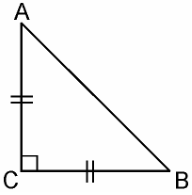# ABC is an isosceles triangle right angled at C. Prove that AB2 = 2AC2 .

ΔABC is an isosceles triangle right angled at C.From ΔACB, ∠C = 90°

AC = BC (By isosceles triangle property)

AB2 = AC2 + BC2 [By Pythagoras theorem]

= AC2 + AC2 [Since, AC = BC]

AB2 = 2AC2